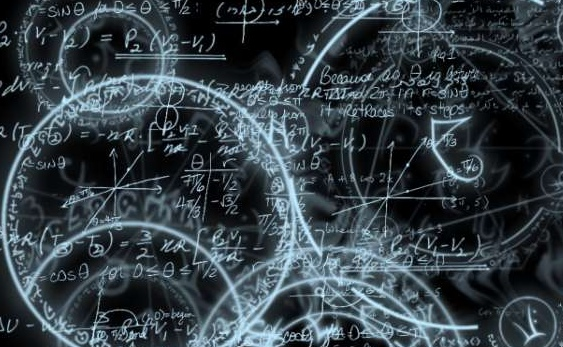## Welcome to H3 Maths

Blog Support for Growing Mathematicians

### Calculus – the language of the stars!

June9“Mathematics is the language of the stars! It was Sir Isaac Newton who worked out a way to mathematically calculate the changing motion of the planets, etc.

Sir Isaac Newton, when pondering the motions of Halley’s Comet, came to the realization that the math that had been used thus far to describe physical motion of massive bodies, simply would not suffice if we were to ever understand anything beyond that of our seemingly limited celestial nook.In a show of pure brilliance … Newton developed the Calculus in which he was able to accurately model the motion of not only Halley’s comet, but also any other heavenly body that moved across the sky.

In one instant, our entire universe opened up before us, unlocking almost unlimited abilities for us to converse with the cosmos as never before. Newton also expanded upon what Kepler started. Newton recognized that Kepler’s mathematical equation for planetary motion, Kepler’s 3rd Law ( P2=A3 ), was purely based on empirical observation, and was only meant to measure what we observed within our solar system. Newton’s mathematical brilliance was in realizing that this basic equation could be made universal by applying a gravitational constant to the equation, in which gave birth to perhaps one of the most important equations to ever be derived by mankind; Newton’s Version of Kepler’s Third Law.” Read more on the fascinating links between Mathematics and Astronomy here

by posted under Uncategorized | Comments Off on Calculus – the language of the stars!